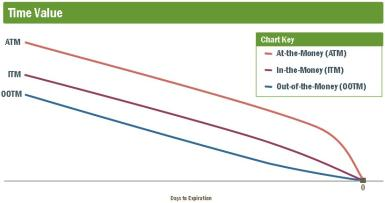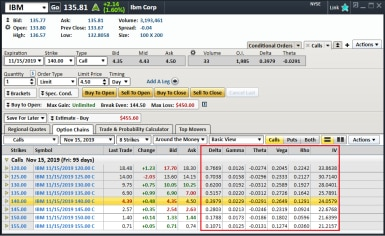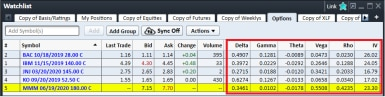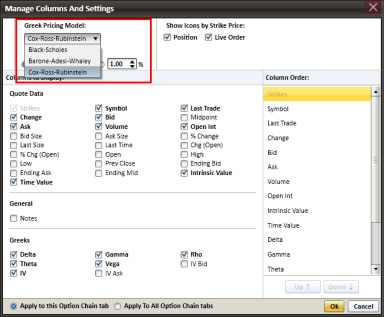Get to Know the Option GreeksKey Points

• Option Greeks measure the different factors that affect the price of an option contract.

• We'll explore the key Greeks: Delta, Gamma, Theta, Vega and Rho.

Options traders often invoke the “Greeks.” What are they, and more importantly, what can they do for you?

In short, the Greeks refer to a set of calculations you can use to measure different factors that might affect the price of an options contract. With that information, you can make more informed decisions about which options to trade, and when to trade them.

They are:

• Delta, which can help you gauge the likelihood an option will expire in-the-money (ITM), meaning its strike price is below (for calls) or above (for puts) the underlying security’s market price.
• Gamma, which can help you estimate how much the Delta might change if the stock price changes.
• Theta, which can help you measure how much value an option might lose each day as it approaches expiration.
• Vega, which can help you understand how sensitive an option might be to large price swings in the underlying stock.
• Rho, which can help you simulate the effect of interest rate changes on an option.

Now that you’ve been introduced, we can explore these calculations in more detail.

Delta

Delta measures how much an option’s price can be expected to move for every \$1 change in the price of the underlying security or index. For example, a Delta of 0.40 means the option’s price will theoretically move \$0.40 for every \$1 change in the price of the underlying stock or index. As you might guess, this means the higher the Delta, the bigger the price change.

Traders often use Delta to predict whether a given option will expire ITM. So, a Delta of 0.40 is taken to mean that at that moment in time, the option has about a 40% chance of being ITM at expiration. This doesn’t mean higher-Delta options are always profitable. After all, if you paid a large premium for an option that expires ITM, you might not make any money.

You can also think of Delta as the number of shares of the underlying stock the option behaves like. So, a Delta of 0.40 suggests that given a \$1 move in the underlying stock, the option will likely gain or lose about the same amount of money as 40 shares of the stock.

Call options

• Call options have a positive Delta that can range from 0.00 to 1.00.
• At-the-money options usually have a Delta near 0.50.
• The Delta will increase (and approach 1.00) as the option gets deeper ITM.
• The Delta of ITM call options will get closer to 1.00 as expiration approaches.
• The Delta of out-of-the-money call options will get closer to 0.00 as expiration approaches.

Put options

• Put options have a negative Delta that can range from 0.00 to –1.00.
• At-the-money options usually have a Delta near –0.50.
• The Delta will decrease (and approach –1.00) as the option gets deeper ITM.
• The Delta of ITM put options will get closer to –1.00 as expiration approaches.
• The Delta of out-of-the-money put options will get closer to 0.00 as expiration approaches.

Gamma

Where Delta is a snapshot in time, Gamma measures the rate of change in an option’s Delta over time. If you remember high school physics class, you can think of Delta as speed and Gamma as acceleration. In practice, Gamma is the rate of change in an option’s Delta per \$1 change in the price of the underlying stock.

In the example above, we imagined an option with a Delta of .40. If the underlying stock moves \$1 and the option moves \$.40 along with it, the option’s Delta is no longer 0.40. Why? This \$1 move would mean the call option is now even deeper ITM, and so its Delta should move even closer to 1.00. So, let’s assume that as a result the Delta is now 0.55. The change in Delta from 0.40 to 0.55 is 0.15—this is the option’s Gamma.

Because Delta can’t exceed 1.00, Gamma decreases as an option gets further ITM and Delta approaches 1.00. After all, there’s less room for acceleration as you approach top speed.

Theta

Theta tells you how much the price of an option should decrease each day as the option nears expiration, if all other factors remain the same. This kind of price erosion over time is known as time decay.

Time-value erosion is not linear, meaning the price erosion of at-the-money (ATM), just slightly out-of-the-money, and ITM options generally increases as expiration approaches, while that of far out-of-the-money (OOTM) options generally decreases as expiration approaches.

Time-value erosionSource: Schwab Center for Financial Research

Vega

Vega measures the rate of change in an option’s price per one-percentage-point change in the implied volatility of the underlying stock. (There’s more on implied volatility below.) While Vega is not a real Greek letter, it is intended to tell you how much an option’s price should move when the volatility of the underlying security or index increases or decreases.

• Volatility is one of the most important factors affecting the value of options.
• A drop in Vega will typically cause both calls and puts to lose value.
• An increase in Vega will typically cause both calls and puts to gain value.

Neglecting Vega can cause you to potentially overpay when buying options. All other factors being equal, when determining strategy, consider buying options when Vega is below “normal” levels and selling options when Vega is above “normal” levels. One way to determine this is to compare the historical volatility to the implied volatility. Chart studies for both values are available on StreetSmart Edge®.

Rho

Rho measures the expected change in an option’s price per one-percentage-point change in interest rates. It tells you how much the price of an option should rise or fall if the risk-free interest rate (U.S. Treasury-bills)* increases or decreases.

• As interest rates increase, the value of call options will generally increase.
• As interest rates increase, the value of put options will usually decrease.
• For these reasons, call options have positive Rho and put options have negative Rho.

Consider a hypothetical stock that’s trading exactly at its strike price. If the stock is trading at \$25, the 25 calls and the 25 puts would both be exactly at the money. You might see the calls trading at, say, \$0.60, while the puts could be trading at \$0.50. When interest rates are low, the price difference between puts and calls will be relatively small. If interest rates increase, the gap will get wider—calls will become more expensive and puts will become less so.

Rho is generally not a huge factor in the price of an option, but should be considered if prevailing interest rates are expected to change, such as just before a Federal Open Market Committee (FOMC) meeting.

Long-Term Equity AnticiPation Securities® (LEAPS®) options are far more sensitive to changes in interest rates than are shorter-term options.

Implied volatility: like a Greek

Though not actually a Greek, implied volatility is closely related. Implied volatility is a forecast of how volatile an underlying stock is expected to be in the future—but it’s strictly theoretical. While it’s possible to forecast a stock’s future moves by looking at its historical volatility, among other factors, the implied volatility reflected in the price of an option is an inference based on other factors, too, such as upcoming earnings reports, merger and acquisition rumors, pending product launches, etc.

Key points to remember:

• Figuring out exactly how volatile a stock will be at any given time is difficult, but looking at implied volatility can give you a sense of what assumptions market makers are using to determine their quoted bid and ask prices. As such, implied volatility can be a helpful proxy in gauging the market.
• Higher-than-normal implied volatilities are usually more favorable for options sellers, while lower-than-normal implied volatilities are more favorable for option buyers, because volatility often reverts back to its mean over time.
• Implied volatility is often provided on options trading platforms because it is typically more useful for traders to know how volatile a market maker thinks a stock will be than to try to estimate it themselves.
• Implied volatility is usually not consistent for all options of a particular security or index and will generally be lowest for at-the-money and near-the-money options.

StreetSmart Edge® has charting studies for historical volatility and implied volatility. By comparing the underlying stock’s implied volatility to the historical volatility, you can sometimes get a good sense of whether an option is priced higher or lower than normal.

Putting Greeks to work

StreetSmart Edge allows you to view streaming Greeks in the options chain of the trading window and in your watch lists. Here is what it looks like.

Streaming Greeks in the trading windowSource: StreetSmart Edge

Streaming Greeks in a watch listSource: StreetSmart Edge

You can arrange the columns to display in any order you like. And, as shown below, you can choose between three of the most widely used pricing models. In addition, the dividend yield and 90-day T-bill interest rate are already filled in. You can use these values or specify your own.

Choose from three widely used pricing modelsSource: StreetSmart Edge

*The values of “risk-free” U.S. Treasury bills fluctuate due to changing interest rates or other market conditions and investors may experience losses with these instruments.